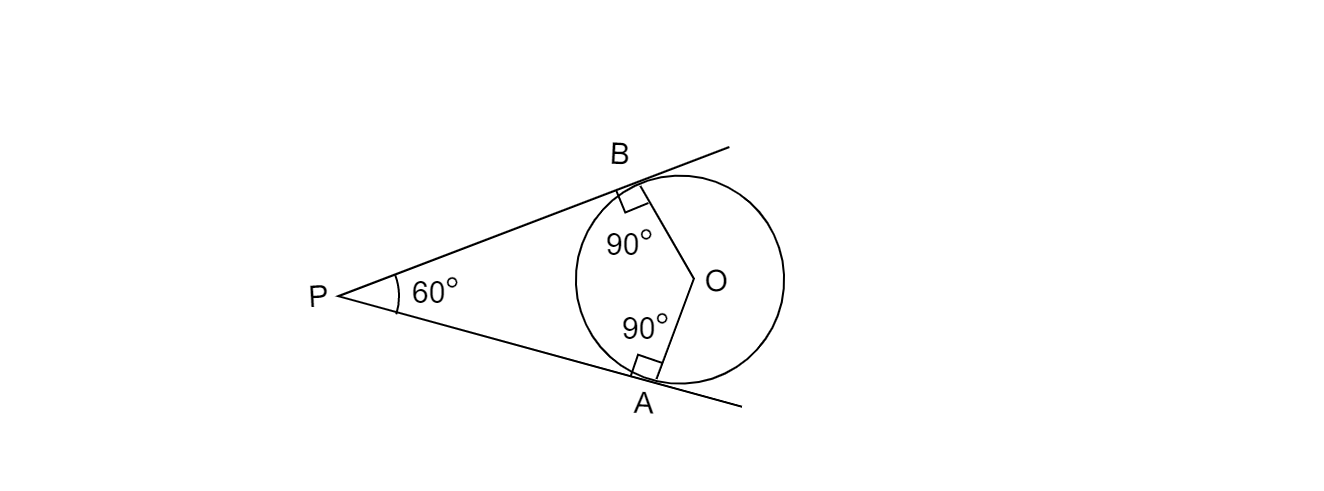# Draw a pair of tangents to a circle of radius 5 cm which are inclined to each other at an angle of $60^o$.

Given:

Radius of the circle 5 cm.

To do:

We have to draw a pair of tangents to the circle inclined to each other at the angle of $60^{o}$.

Solution:Steps of construction:.

1. Draw a circle of radius 5 cm with centre O.

2. Here, tangents are inclined at an angle of $60^{o}$ to each other. If PA and PB are the required tangents to the circle, then$\angle APB=60^{o}$ and AOBP is a cyclic quadrilateral, then $\angle AOB=180^{o} -60^{o} =120^{o}.$

3.Take a point A on the circumference of the given circle and draw an angle of $120^o$ to OA on point O such that $\angle AOB=120^{o}$. B is another point where $\angle AOB=120^{o}$ intersects the circle.

4.Draw a perpendicular to OA.

5.Draw a perpendicular to OB.

6.perpendiculars on A and B intersects on point P.

Hence PA and PB is the required  pair of tangents to the circle inclined to each other at the angle of $60^{o}$.